# Charging and Discharging a Capacitor

DC-Circuits > Charging and Discharging a Capacitor

A Capacitor is a passive device that stores energy in its Electric Field and returns energy to the circuit whenever required. A Capacitor consists of two Conducting Plates separated by an Insulating Material or Dielectric. Figure 1 and Figure 2 are the basic structure and the schematic symbol of the Capacitor respectively.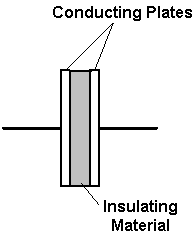Figure 1: Basic structure of the Capacitor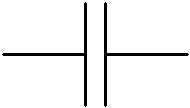Figure 2: Schematic symbol of the Capacitor

When a Capacitor is connected to a circuit with Direct Current (DC) source, two processes, which are called "charging" and "discharging" the Capacitor, will happen in specific conditions.

In Figure 3, the Capacitor is connected to the DC Power Supply and Current flows through the circuit. Both Plates get the equal and opposite charges and an increasing Potential Difference, vc, is created while the Capacitor is charging. Once the Voltage at the terminals of the Capacitor, vc, is equal to the Power Supply Voltage, vc = V, the Capacitor is fully charged and the Current stops flowing through the circuit, the Charging Phase is over.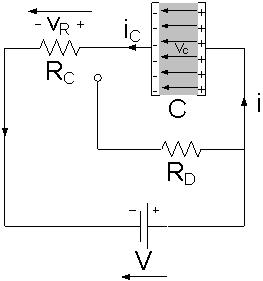Figure 3: The Capacitor is Charging

A Capacitor is equivalent to an Open-Circuit to Direct Current, R = ∞, because once the Charging Phase has finished, no more Current flows through it. The Voltage vc on a Capacitor cannot change abruptly.

When the Capacitor disconnected from the Power Supply, the Capacitor is discharging through the Resistor RD and the Voltage between the Plates drops down gradually to zero, vc = 0, Figure 4.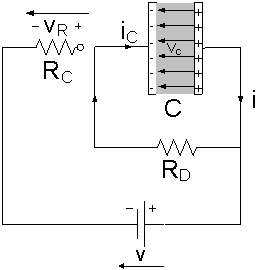Figure 4: The Capacitor is Discharging

In Figures 3 and 4, the Resistances of RC and RD affect the charging rate and the discharging rate of the Capacitor respectively.

The product of Resistance R and Capacitance C is called the Time Constant τ, which characterizes the rate of charging and discharging of a Capacitor, Figure 5.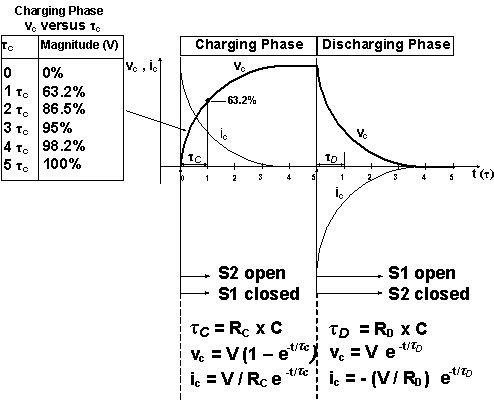Figure 5: The Voltage vc and the Current iC during the Charging Phase and Discharging Phase

The smaller the Resistance or the Capacitance, the smaller the Time Constant, the faster the charging and the discharging rate of the Capacitor, and vice versa.

Capacitors are found in almost all electronic circuits. They can be used as a fast battery. For example, a Capacitor is a storehouse of energy in photoflash unit that releases the energy quickly during short period of the flash.

DC-Circuits > Charging and Discharging a Capacitor